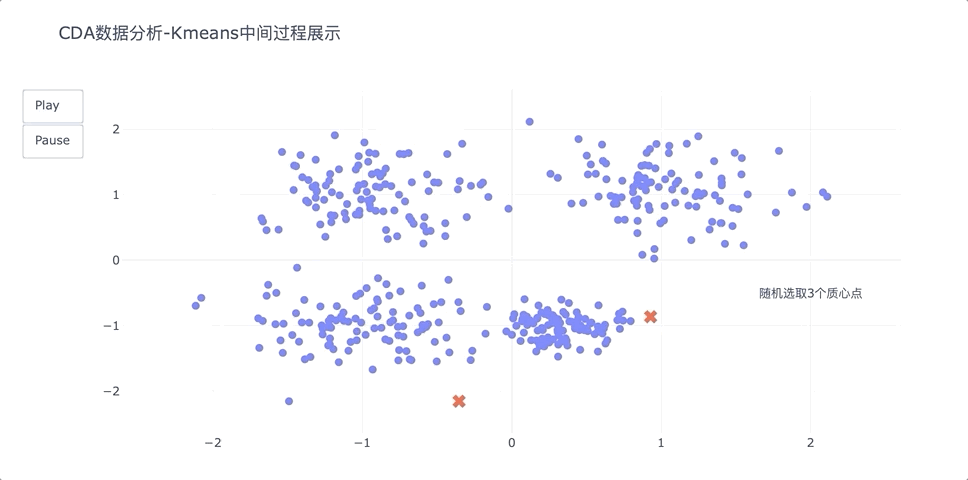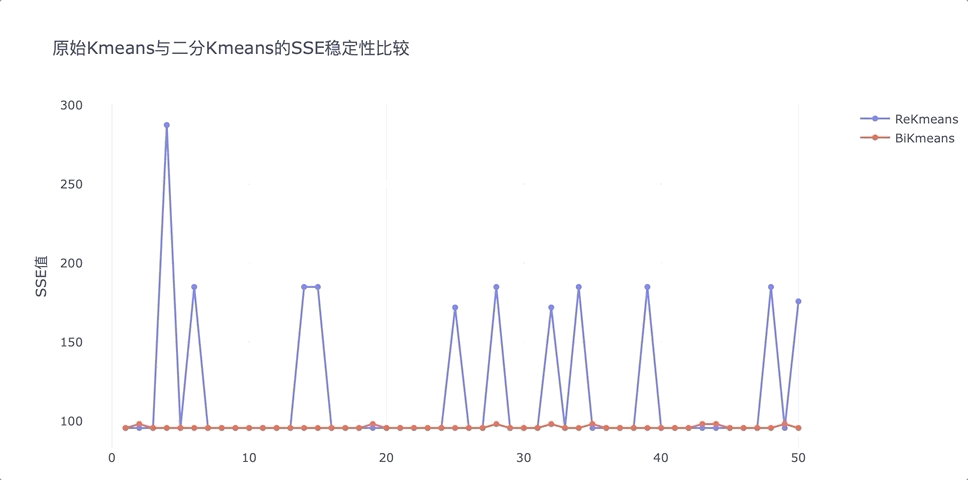##全国校区# Kmeans优化算法：二分K-means聚类算法2020-05-29

## 算法的理解

Bi这里是的意思就是Binary，二进制的意思，所以有时候叫这个算法为二进Kmeans算法。为什么我们需要用BiKmeans呢，就是为了解决初始化k个随机的质心点时其中一个或者多个点由于位置太极端而导致迭代的过程中消失的问题。BiKmeans只是Kmeans其中一个优化方案，其实还是有很多优化的方案，这里BiKmeans容易讲解和理解，并且容易用numpy, pandas实现。

1. 使用Kmeans(k=2)将数据集分成2个簇，记录SSE
2. 对于每一个簇来说，都有自己当前的SSE，取名为父节点SSEa. 对这些簇都进行Kmeans二分类，并且记录分出的2个簇的SSE只和，称之为子节点总SSEb. 记录这个簇被2分类之后SSE的差值，SSE差值 = 父节点SSE - 子节点SSE
3. 选择SSE差值最大的那个簇进行划分，而其他的簇不进行划分。
4. 重复第二的步骤，直到簇的总个数达到K

## 用决策树的方法理解BiKmeans

1. 使用Kmeans对根节点做2分类。得出2个簇，簇内SSE分别为40和30，也就是说如果我们对整体数据集做一次Kmeans二分类的话，我们的整体SSE会下降100-(30+40)=301. 在此时可以对每个叶节点的数据集进行二分类，查看这个簇做二分Kmeans后，SSE下降多少。从这里可以看出，对簇1来说，SSE的变化为40-(20+15)=5，对簇2来说，SSE的变化为30-(10+10)=10。所以说对这2个簇来说的话，应该保留簇1，对簇2进行二分Kmeans。这样的话可以最大程度的减少总体SSE，因为簇2二分Kmeans后SSE下降的最快。结果就是以下的情况，当前叶节点有3个，也就是说当前我们有3个簇，还没有达到我们的目标，4个簇，继续对每个叶节点进行划分。注，这里原来的簇2就不复存在了，变成了小一点的簇2和簇3。1. 从上面的图看出，现在有3个簇，对每个簇都做二分Kmeans，之前簇1的SSE差值已经算过了，是40-(20+15)=5，对于簇2来说，SSE变化为10-(8+1)=1, 对于簇3来说，SSE的变化为10-(7+1)=2。这里簇1的SSE变化值最大，优先对簇1做二分Kmeans，得出以下结果。1. 当前决策树一共有4个叶节点，每个叶节点都代表一个簇，簇的个数已经达到我们的目标。算法结束。

## 代码实现

from Kmeans_pack import *

r = 4
k = 3
x , y = make_blobs(n_samples = 400,
cluster_std = [0.2, 0.4, 0.4, 0.4],
centers = [[0.3,-1],[1,1],[-1,1], [-1,-1]],
random_state = r
)
np.random.seed(r)
sim_data = pd.DataFrame(x, columns = ['x', 'y'])
sim_data['label'] = y
dataset = sim_data.copy()\left[ \begin{matrix} (x_{1}-\bar{x})^2 + (y_{1}-\bar{y})^2 \\ (x_{2}-\bar{x})^2 + (y_{2}-\bar{y})^2 \\ (x_{3}-\bar{x})^2 + (y_{3}-\bar{y})^2 \end{matrix} \right]⎣⎡(x1−xˉ)2+(y1−yˉ)2(x2−xˉ)2+(y2−yˉ)2(x3−xˉ)2+(y3−yˉ)2⎦⎤

1. 用的方法就是使用np.power(data - mean, 2).sum(axis = 1)得出的就是这个簇一开始的SSE。
2. 将数据集带入之前写的Kmeans_regular后设置k=2，会给出SSE_list, SSE_list[-1]会给出Kmeans聚类好数据集之后2个簇加起来SSE的综合，并且也会给出curr_group, 用来划分我们的簇，方便选取其中的簇带入下一次迭代
def Split(dataset):
#假设当前数据不是第一次二分Kmeans，就是说传进来的是整体的数据集，当前的质心点就是每个特征的平均值
temp_data = dataset.loc[:, dataset.columns != 'label'].copy()

#计算当前数据集的SSE
current_error = np.power(temp_data - temp_data.mean(), 2).sum().sum()

#对数据集做二分Kmeans
curr_group, SSE_list, centers = Kmeans_regular(temp_data, k = 2)

#记录二分后的SSE
after_split_error = SSE_list[-1]

#已经有了curr_group将二分类后的数据集先拿出来
clusters = list(dataset.groupby(curr_group))

#这里有非常非常少的情况会出现二分Kmeans中初始的质心点其中一个由于离所有的都太远，导致丢失的情况
#所以这里多加了一个判断，假如其中一个质心掉了，那上面给的clusters只有一个而不是两个
#在这个情况下，dataset没有被成功二分类，我们需要将dataset自身给return，进行下一次迭代，肯定有一次迭代能成功分出2个簇
#所以在这个情况下entropy就是current_error, cluster1就是dataset自己，cluster2为空
if len(clusters) == 1:
entropy = current_error
return [entropy, dataset, None, current_error]

#分别取出2个簇
cluster1, cluster2 = [i for i in clusters]

#计算这个簇做了二分Kmeans后SSE下降的多少
entropy = current_error - after_split_error

#最后返回结果，返回当前这个簇二分后的SSE差值，分出的簇1和簇2，还有当前这个簇的SSE
return [entropy, cluster1, cluster2, current_error]


entropy, cluster1, cluster2, current_error = Split(dataset)
entropy, cluster1.shape, cluster2.shape, current_error

(432.9176440191153, 200, 200, 813.3842612925762)


def bi_iterate(dataset, k = 4):
#首先准备一个空的cluster_info_list,这个list是用来存二分后的结果，里面每一个元素都是一个簇
#对于每个元素来说，它代表的是个簇，里面记录的这个簇的[entropy, cluster1, cluster2, current_error]
#也就是每个簇的[SSE差值，第一个二分后的簇，第二个二分后的簇，当前簇的SSE]
cluster_info_list = []

#使用while做循环直到cluster_info_list里面一共达到k个簇的时候停止
while len(cluster_info_list) < k:
#假如当前我们是第一次迭代的话也就是cluster_info_list是空list的话做以下操作
if len(cluster_info_list) == 0:

#直接用Split函数，将整体数据集放入cluster_info_list里，然后下面的操作都不用，continue进入下一个循环
cluster_info_list.append(Split(dataset))
continue

#首先将cluster_info_list最后一个元素取出来，cluster_info_list里面是所有簇的信息
#我们后面会对cluster_info_list做sort，由于cluster_info_list里面每个元素的第一位是SSE差值
#所以我们做完sort后，最后一个元素肯定是SSE差值(entropy)最大的那一个，也就是我们需要下一步做二分的簇
#将最后一个元素里的2个clusters取出来后，将这个当前在cluster_info_list里SSE最大的一个簇删除掉(pop方法)
#取而代之的是Split(cluster1)和Split(cluster2)，也是就尝试着对新的两个cluster尝试去算SSE差值

cluster1, cluster2 = cluster_info_list[-1][1:-1]
cluster_info_list.pop(-1)

#将Split(cluster1)和Split(cluster2)的结果追加到cluster_info_list
#注意假如只有cluster2给出的是None，则碰到二分类不成功的情况，cluster1还为原来上一次dataset，cluster2为空
#不将cluster2追加到cluster_info_list当中
cluster_info_list.append(Split(cluster1))
if cluster2 is not None:
cluster_info_list.append(Split(cluster2))

#整体的cluster_info_list进行一次sort，找出当前所有的簇中，哪一个簇的SSE差值最大
#这里我们是需要对整体cluster_info_list做sort，因为新追加进去的2个心cluster的SSE差值可能没有cluster_info_list里已经记录的簇的SSE大。
cluster_info_list.sort()

#进入下一个循环
return cluster_info_list


from Kmeans_pack import *

def Split(dataset):
temp_data = dataset.loc[:, dataset.columns != 'label'].copy()
current_error = np.power(temp_data - temp_data.mean(), 2).sum().sum()
curr_group, SSE_list, centers = Kmeans_regular(temp_data, k = 2)
after_split_error = SSE_list[-1]
clusters = list(dataset.groupby(curr_group))
if len(clusters) == 1:
entropy = current_error
return [entropy, dataset, None, None, current_error]
cluster1, cluster2 = [i for i in clusters]
entropy = current_error - after_split_error
return [entropy, cluster1, cluster2, centers, curr_group, current_error, dataset]

def bi_Kmeans(dataset, k = 4):
cluster_info_list = []
while len(cluster_info_list) < k:
if len(cluster_info_list) == 0:
cluster_info_list.append(Split(dataset))
continue
cluster1, cluster2 = cluster_info_list[-1][1:3]
cluster_info_list.pop(-1)
cluster_info_list.append(Split(cluster1))
if cluster2 is not None:
cluster_info_list.append(Split(cluster2))
cluster_info_list.sort()
return cluster_info_list


np.random.seed(1)
cluster_info_list = bi_Kmeans(dataset, k = 4)


## ReKmeans和BiKmeans的结果对比

regular_SSE = []
bi_SSE = []
for i in range(50):
curr_group, SSE_list, centers = Kmeans_regular(dataset, k = 4)
cluster_info_list = bi_Kmeans(dataset, k = 4)
bi_sse = sum([i[-1] for i in cluster_info_list])
regular_SSE.append(SSE_list[-1])
bi_SSE.append(bi_sse)

data_compare = pd.DataFrame({'ReKmeans':regular_SSE,'BiKmeans':bi_SSE})
data = [go.Scatter(x = data_compare.index + 1,
y = data_compare[i],
mode = 'lines+markers',
name = i
) for i in data_compare.columns]

layout = go.Layout(title = '原始Kmeans与二分Kmeans的SSE稳定性比较',
xaxis = {'title' : '次数'},
yaxis = {'title' : 'SSE值'},
template = 'plotly_white'
)

fig = go.Figure(data = data, layout = layout)
#fig.show()## BiKmeans大致中间过程OK